# Fleas!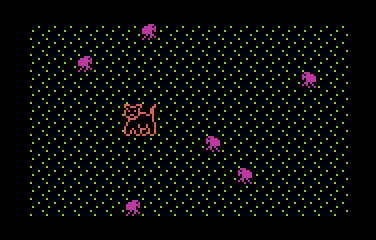This is a little game for the 2016 NOMAM's BASIC 10-liners Contest. This program fits in the PUR-120 category, and it was written using TurboBASIC XL 1.5 for the 8-bits ATARI XL/XE. Development started on 2015-08-06, and it took 4 days. The final version's date is 2016-03-14.

UPDATE: It obtained the 4th place of 22 entries in the category.

## Description

Keep Doggy without fleas. Each round has more fleas. There are only three rounds.

## Instructions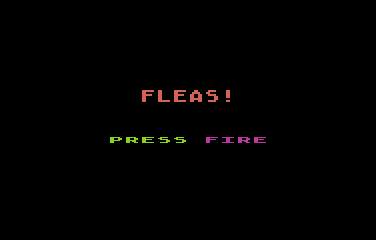Press joystick's button to start.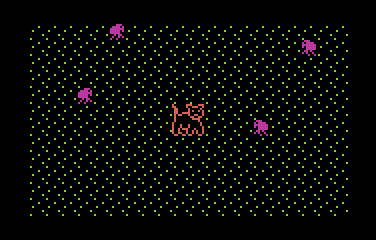Move the dog around the garden trying to not be cought by a flea. You cannot move by the edge. Round 1 has 4 fleas.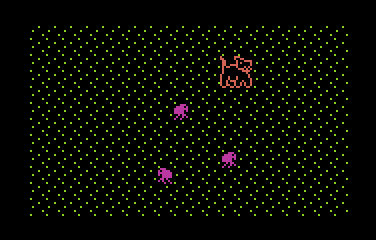When Doggy is bitten by a flea, the round ends.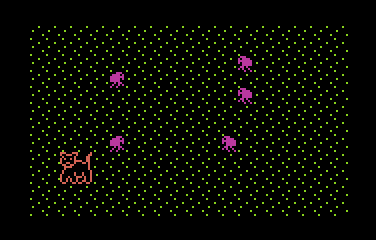Round 2 is a bit slower than the previous one, but has 5 hungry fleas. Keep moving around!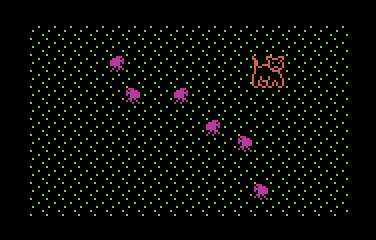Round 3 has 6 fleas lurking.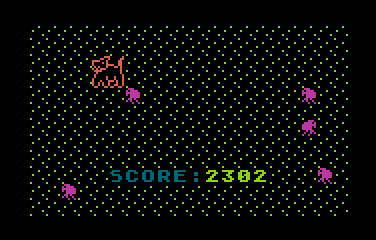The game is over when the 3rd bite of a flea occurs. The score will be greater the longer you stay without being bitten.

## Development of the game

I'd read about NOMAM's tenliners contest some years ago and thought it would be an interesting challenge. But, what could be done in just 10 lines of BASIC code? I tried some of the published games and discovered few jewels. Those were written in TurboBASIC XL. But I also noted that listings had 10 lines of very long size. But that was OK, because abbreviations were allowed to enter the code into BASIC. 120 chars are the maximum length of a logical line in the screen editor of Atari 8bit machines, that is, 3 physical lines. Also, unnecesary spaces could also be removed as long as the parser could recognize the syntax. Variable names of just one character also save space.

I've never programmed in TurboBASIC XL before, so I had no references about the new instructions, specially the flow control ones: WHILE-WEND, REPEAT-UNTIL and IF-ELSE-ENDIF. Other instructions like DPOKE, functions like DPEEK() and RAND() and operators like DIV and MOD allowed to omit unnecesary algebra to perform a single step, thus reducing the number of instructions and bytes in the listing. Also, MOVE and -MOVE are the key instructions to manage memory quickly enough to animate things on screen, redefine Player/Missile data, and many other uses.

With all that in mind, I thought which of the little games I liked could be written using few BASIC instructions, and one of them was "Shooting Stars", a M/L game published in 1984 by A.N.A.L.O.G magazine, which I modded a little and renamed as "Wow!" for personal use. However, I already was programming a game in assembler which I sent ABBUC's 2015 Game Contest, so I didn't do anything this time for NOMAM's. But when that project was finished, I reconsidered it, mainly because I knew that another chilean programmer won 2015 edition of NOMAM in PUR-120 category.

I sat down on August the 1st and started writing a character version of "Wow!" in graphisc mode 2+16 (big font without text box). After a couple of hours, I had a working code as a proof of concept: a letter that could be moved through the screen with a joystick trying to avoid a hit by any of the 6 numbers bouncing around. Instead of a constantly increasing speed (which I couldn't give because it was BASIC!), I decided to change the the amount of numbers on screen: start with 4 bouncing numbers, the add an extra number for the 2nd life, and another one for the 3rd and last life. I called this game as "Alphabetics versus Numerics", or "AlphaNumeric" for short, as each life was a different letter: A, B and C, and the numbers were from 1 to 4, 5 and 6, respectively.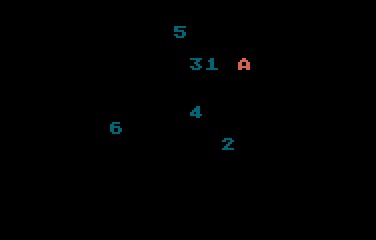Proof of concept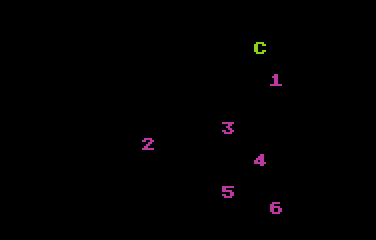1st version of AlphaNumeric

The programming was done in a text editor, where I could copy the code into the buffer and paste it in Altirra for testing. None of the coding was done directly in the emulator, because logical lines expanded to more than 120 chars. Yes, I was writing the BASIC code directly in abbreviated form. That gave me an idea of the ammount of space I've already used and what more I could do. But I found some restrictions: some instructions required line numbers, like GOTO, and others required the remaining space of a line like IF-THEN and DATA. Those tie down on how 10 lines could be used.

Technically speaking, the screen manipulation is done using POKE to put elements on it and PEEK to check for collisions, while arrays are used only to keep current position of each element on screen and the current moving direction on each axis. The initial position and direction of each number were randomly calculated. Other arrays are used to turn joystick position into moving deltas on each axis. A simple formula is required to turn coordinates into screen memory positions.

But the game was too easy: sometimes you could find blind-points, i.e. places in screen that would never be reached by numbers. I decided to turn the letter into a 2x2 squad of letters. AB/CD, EF/GH and IJ/KL were the squads for each of the 3 rounds. Minor changes were needed for this new version of the game, and it still used less than 8 lines of code (now, I think it could fit PUR-80 category!). Then, I noticed that sometimes two numbers appeared in the same position and going into the same direction, so some validations should also be added at the round set up. DPEEK and DPOKE were used to move the squad, while PEEK and POKE remained for the numbers. An asterisk was used to show the collision. I was satisfied with the result, and had an entry for the next contest.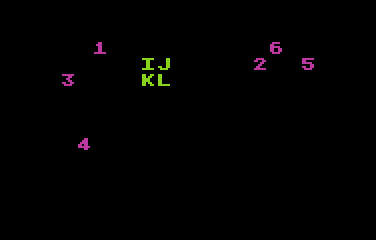2nd version of AlphaNumeric

Another day, I thought it would be nice to change the fonts and turn the game into a graphical one instead of a texted one. I had enougth space to add the font definition for the few game characters. Many ideas came to my mind, like some flying saucers surrounding a mothership or such, but I preferred the dog avoiding the fleas. And it also simplified some code, because the squad and the bouncing soldiers were now always the same. Instead of a collision mark, I added a growling face to the dog. Finally, I added a pattern for the playfield to show the screen limits. It was a pattern because a solid one would show squares where a character is, instead we are using our mind's ability to fill the areas and get a better idea of what we are seen. Obviously, I had to change the name of the game, and "Fleas!" was a proper one.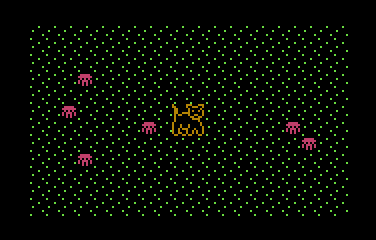1st version of Fleas!

Today, more than 7 months later, I reviewed the code to describe it here, and I was surprised... I used some of the usual Atari BASIC statements like GOTO to control the flow, and some other algorithms that waste valuable listing space. So, I decided to change some of that code in order to use all what I've learnt after 5 tenliners in my production list. But this time, instead of to write the code using abbrevations, I used the tools I developed later, so the listing was much more clear and I was able to use more tricks.

The revised version saved more space, so I could add more fonts for the "sprites" to create a sort of animation. First, fleas were modified to show where are they going to. Then I did the same with Doggy. Also, changed the growling head that appears when a flea gets into Doggy's hair, because it seemed it was barking, which didn't match the sound.2nd version of Fleas!

Get the FLEAS.ATR file and set it as drive 1 in a real Atari or emulator. Turn on the computer and the game should start after loading. A joystick in port 1 is required.

## The code

The abbreviated BASIC code is the following: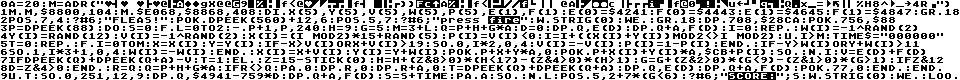The full and expanded BASIC listing is:

 ```a=20 ``` Just a constant used to replace that number that repeats in many places and then to save some bytes in the listing. ```m=adr("binary data") ``` Bitmap for the characters. ```move m,\$8800,104 move \$E068,\$8868,408 ``` Copies the character bitmaps into RAM and appends font bitmaps to them. ```dim x(5),y(5),v(5),w(5),p(5),e(1),f(1) ``` Defines the required arrays: x(0-5): Horizontal coordinate of every flea. y(0-5): Vertical coordinate of every flea. v(0-5): Horizontal speed of every flea, may be 1 to the right or -1 to the left. w(0-5): Vertical speed of every flea, may be 1 to the bottom or -1 to the top of the screen. p(0-5): Horizontal direction of every flea, used to select the character to display: 0 if going to the right, 1 to the left. e(0-1): Top half of Doggy, depending if going to the right (0) or to the left (1). f(0-1): Bottom half of Doggy, depending on the direction as above. ```e(0)=\$4241 f(0)=\$4443 e(1)=\$4645 f(1)=\$4847 ``` Sets the words (2 bytes) for every half of Doggy, based on the horizontal direction. ```graphics 18 ``` Sets graphics mode 2+16 (big fonts without text box). ```position 7,4 ? #6;"FLEAS!" ``` Prints the name of the game in the title screen. ```poke dpeek(560)+12,6 ``` Changes one line to single resolution (graphics mode 1). ```position 5,7 ? #6;"PRESS FIRE" ``` Prints the instruction in the flat line. ```while strig(0) wend ``` Waits until joysticks button is pressed. ```graphics 18 ``` Reset the playfield to standard graphics mode 2 texts. ```dpoke 708,\$28CA ``` Changes two color registers. ```poke 756,\$88 ``` Enables the new charset in RAM. Now, the "space" has green dots, so the playfield looks like a garden. ```p=dpeek(88) ``` Memory address of the upper left corner of the playfield. ```do ``` Game loop. The game starts here. ``` s=0 ``` Reset the score. ``` for l=0 to 2 ``` Iterates 3 times, each cycle is a round. ``` -move p+1,p,240 ``` Cleans the screen by copying and propagating the first byte from outside the playfiels into it. ``` h=9 g=5 ``` Initial coordinate of the upper left byte of Doggy. Column 9 horizontally and row 5 vertically. ``` m=3+l ``` Sets the number of fleas in the round. ``` q=p+h+g*a ``` Calculates the position in memory of Doggy. ``` d=0 ``` Sets the initial direction of Doggy as going to the right. ``` dpoke q,e(d) dpoke q+a,f(d) ``` Puts Doggy in the playfield. ``` i=0 repeat ``` Routine to set-up the initial position of every flea in the round. ``` w(i)=-1^rand(2) ``` Randomly sets the initial horizontal direction of a flea. ``` y(i)=rand(12) ``` Randomly sets the initial vertical position of a flea. ``` v(i)=-1^rand(2) ``` Randomly sets the initial vertical direction of a flea. ``` x(i)=(i mod 2)*15+rand(5) ``` Ramdomly sets the initial horizontal position of a flea, but half of them start on the left, and half on the right side of the screen. ``` p(i)=v(i)<0 ``` Sets the initial direction of a flea as required by the font to display the proper flea. ``` i=i+((x(i)+y(i)) mod 2 <> i mod 2) ``` Try to ensure that half of the fleas moves on "white" diagonals and the other half on "black" diagonals as if the playfield were a chess table. ``` until i>m ``` Repeat for every flea of the round. ``` time\$="000000" ``` Resets the internal timer. ``` t=0 ``` T is the collision register between Doggy and the fleas. ``` repeat ``` Round loop... ``` for i=0 to m ``` Routine to move the fleas: ``` x=x(i) y=y(i) ``` Selects the coordinates of the flea. ``` if -x>v(i) or x+v(i)>19 ``` If it has to bounce on one of the sides: ``` sound 0,i*2,0,4 ``` Starts a bounce sound. The pitch is different for each flea ``` v(i)=-v(i) ``` Changes the horizontal direction of the flea. ``` p(i)=1-p(i) ``` Turns over it's character. ``` endif ``` End of horizontal bounce routine. ``` if -y>w(i) or y+w(i)>11 ``` If it has to bounce on the top or the bottom of the screen: ``` sound 1,i*3+1,0,4 ``` Plays a sound. Each flea has a different pitch. As it uses different sound channels for vertical and horizontal bounces, if it bounces in a corner, a double sound should be heard. ``` w(i)=-w(i) ``` Changes the vertical direction to the other side. ``` endif ``` End of the vertical bounce routine. ``` x(i)=x+v(i) y(i)=y+w(i) ``` Calculates the new coordinates of a flea. ``` poke p+x+y*a,0 ``` Removes the flea from it's old location. ``` poke p+x(i)+y(i)*a,\$CB+p(i) ``` Puts the flea into its new location. ``` sound ``` Turns of the sound of all bounces, if any! ``` next i ``` End of the flea's moving routine. ``` v=e(d)+f(d) ``` Value of the current shape of Doggy. ``` if dpeek(q)+dpeek(q+a)-v ``` If the memory locations of the playfield where Doggy is does not have the same value, it's because a flea hit Doggy. ``` t=1 ``` Sets the collision register. ``` else ``` If Doggy was not hit by a flea, it could be moved by the player. ``` z=15-stick(0) ``` Read the joystick position and negates al the bits, so a 1 means that it was moved in a given direction. ``` h=h+(z&8>0)*(h<17)-(z&4>0)*(h>1) ``` Changes the horizontal position of Doggy if any of the horizontal bits of the joystick was set and Doggy is not going to the border of the playfield. Only the fleas can go to the border where they bounce. ``` g=g+(z&2>0)*(g<9)-(z&1>0)*(g>1) ``` The same for the vertical position of Doggy. ``` if z&12 ``` If Doggy was moved horizontally: ``` d=z&4>0 endif ``` Sets the direction flag, that is used to display Doggy walking to the right or the left. ``` r=q ``` Saves the current location of Doggy in the playfield. ``` q=p+h+g*a ``` Calculates the new location. ``` if r<>q ``` If the lacation is not the same: ``` pause 0 ``` Waits for a Vertical Blank to avoid glitches. ``` dpoke r,0 dpoke r+a,0 ``` Remove Doggy from the playfield. ``` t=dpeek(q)+dpeek(q+a) ``` Checks the memory for the new location of Doggy. If it is not empty, there is a collision with a flea. ``` dpoke q,e(d) dpoke q+a,f(d) ``` Puts Doggy in its new location, looking to the left or to the right according to it's current walking direction. ``` poke 77,0 ``` Resets the ATRACT mode counter. This avoids screen colors to change during the game. ``` endif endif ``` End of the moving routine. ``` until t ``` End of the round loop. Quits when there is a collision betwenn Doggy and a flea. ``` sound 0,251,12,9 ``` Plays a growling sound. ``` dpoke q,\$4941-759*d ``` Changes the upper side of Doggy to display it's head showing the teeths in the proper direction. ``` dpoke q+a,f(d) ``` Displays again the bottom side of Doggy to possibly remove from scren a flea that had hit there. ``` s=s+time ``` Increases the score based in the number of "jiffies" (internal clock) since the begin of the round. ``` pause a sound ``` Waits for a moment and turn of the growling sound. ``` next l ``` Goes to the next round, or finish the game if it was the last loop. ``` position 5,2+7*(g<6) ?#6;"SCORE:";s ``` Prints the final score. If Doggy is in the upper half of the playfield, the score is displayed in the bottom half, and viceversa. ``` while strig(0) wend ``` Waits for the joystick's button to start again. ```loop ``` Restart the game loop.